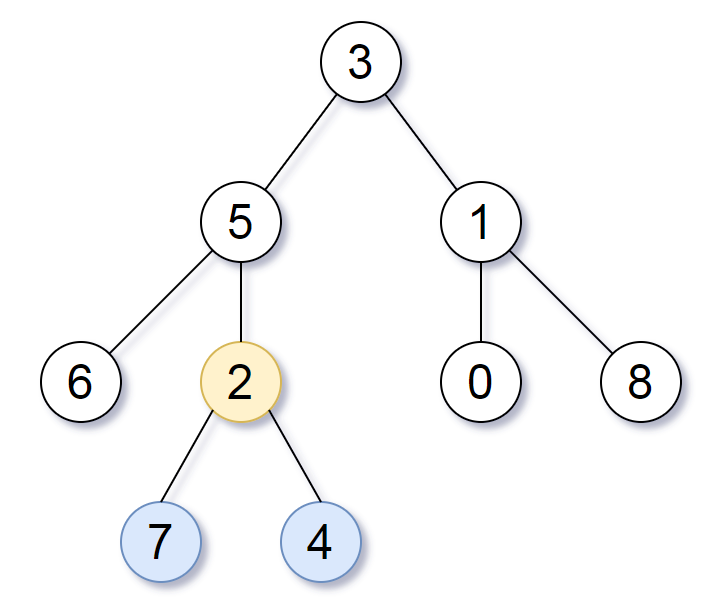## 1123. Lowest Common Ancestor of Deepest Leaves

Given the `root` of a binary tree, return the lowest common ancestor of its deepest leaves.

Recall that:

• The node of a binary tree is a leaf if and only if it has no children
• The depth of the root of the tree is `0`. if the depth of a node is `d`, the depth of each of its children is `d + 1`.
• The lowest common ancestor of a set `S` of nodes, is the node `A` with the largest depth such that every node in `S` is in the subtree with root `A`.

Note: This question is the same as 865: https://leetcode.com/problems/smallest-subtree-with-all-the-deepest-nodes/

Example 1:```Input: root = [3,5,1,6,2,0,8,null,null,7,4]
Output: [2,7,4]
Explanation: We return the node with value 2, colored in yellow in the diagram.
The nodes coloured in blue are the deepest leaf-nodes of the tree.
Note that nodes 6, 0, and 8 are also leaf nodes, but the depth of them is 2, but the depth of nodes 7 and 4 is 3.```

Example 2:

```Input: root = 
Output: 
Explanation: The root is the deepest node in the tree, and it's the lca of itself.
```

Example 3:

```Input: root = [0,1,3,null,2]
Output: 
Explanation: The deepest leaf node in the tree is 2, the lca of one node is itself.
```

Constraints:

• The number of nodes in the tree will be in the range `[1, 1000]`.
• `0 <= Node.val <= 1000`
• The values of the nodes in the tree are unique.

## Rust Solution

``````struct Solution;
use rustgym_util::*;

trait Postorder {
}

fn postorder(&self) -> (usize, TreeLink) {
use std::cmp::Ordering::*;
if let Some(node) = self {
let left = &node.borrow().left;
let right = &node.borrow().right;
let (height_l, left_lca) = left.postorder();
let (height_r, right_lca) = right.postorder();
match height_l.cmp(&height_r) {
Less => (height_r + 1, right_lca),
Greater => (height_l + 1, left_lca),
Equal => (height_l + 1, self.clone()),
}
} else {
(0, None)
}
}
}

impl Solution {
let (_, lca) = root.postorder();
lca
}
}

#[test]
fn test() {
let root = tree!(1, tree!(2), tree!(3));
let res = tree!(1, tree!(2), tree!(3));
assert_eq!(Solution::lca_deepest_leaves(root), res);
let root = tree!(1, tree!(2, tree!(4), None), tree!(3));
let res = tree!(4);
assert_eq!(Solution::lca_deepest_leaves(root), res);
let root = tree!(1, tree!(2, tree!(4), tree!(5)), tree!(3));
let res = tree!(2, tree!(4), tree!(5));
assert_eq!(Solution::lca_deepest_leaves(root), res);
let root = tree!(
1,
tree!(2, tree!(3, None, tree!(6)), tree!(4, None, tree!(5))),
None
);
let res = tree!(2, tree!(3, None, tree!(6)), tree!(4, None, tree!(5)));
assert_eq!(Solution::lca_deepest_leaves(root), res);
}
``````

Having problems with this solution? Click here to submit an issue on github.